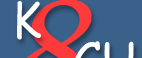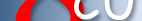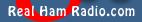Home | Terms of Use | Contact | Community | Guest Book | Privacy | Site Map.
 xEver wonder what the EFC circuitry in your GPS receiver is like? In this article, Joe Geller, KO2Y describes his reverse engineering efforts on the Z3801A DAC and analog circuits that drive the frequency vernier (Vefc) of the HP 10811 dual oven oscillator. For a fundamental review, see Joe's discussion of Z3801A fractional parts. The Z3801A circuitry from the EFC DAC through to the Vefc SMB connector that connects to the HP 10811 voltage controlled oven oscillator has been reverse engineered. This part of the receiver can be divided into two parts, the control of the 16 bit DAC, and the analog circuitry that follows. THE DAC The Z3801A digital firmware develops a 20 bit correction number (EFC integer) that ultimately controls the HP 10811 dual oven oscillator tuning via its Vefc input. The integer is truncated to 16 bits and transferred to a 16 DAC operating between -5 volts and +5 volts. The EFC integer, NEFC, read by the command ":DIAG:ROSC:EFC:ABS?" ranges from 0 to 220 which is 1,048,576. Typically, the HP oscillators are tuned over a range of -5 Volts to +5 Volts, and the DAC is observed by measurement to have a positive Vref of +5V and a negative Vref of -5V. So, this is going to be an offset scale where 0 = -5 Volts and 1,048,575 = +5 Volts. Note that the full scale integer is always 2N-1 because zero is the first used value. The equations corresponding to this relationship are: (NEFC * 9.53675E-06) -5 = VDAC, or (VDAC +5) / 9.53675E-06 = NEFC The percent EFC reported by the Z3801A seems to agree with the above relations: Here -100% = -5 Volts or EFC integer of 0, and +100% = +5 Volts = EFC integer of (220)-1. O% = EFC voltage of 0 Volts or (220-1) - (220/2) = an EFC integer of (220-1) Now the really odd thing about this scale is that it is 200% wide! (-100% to +100%) Here is an an example: The range from 524,288 to 1,048,575 (220-1) is 0% to + 100% or this range is 1,048,575 -524,288 = 524,287. By algebraic ratios then ((EFC number - 524,288) / 524,288)*100% = the EFC % as read by the command ":DIAG:ROSC:EFC:REL?". A Specific Example: As a check I read an EFC number of: 776322 ((776,313- 524,288 / 524,288) *100% = 48.0700, and my Z returns 48.0700 %! Now to check the equation at the end points: for NEFC of 0: ((0 - 524,288 / 524,288) *100% = -100% and for NEFC = 220-1: ((1,048,575 - 524,288 / 524,288) *100% = +100% This section ends at test point 6 (TP6) on the circuit board. TP6 is the output of the Analog Devices AD569 16 bit DAC (AD569 Data Sheet). Measuring the Voltage at TP6 and comparing the measured voltage to the voltage predicted by the equations above yields the first analog errors. Besides the errors introduced by your multimeter, there will be additional errors caused by the DAC and the DAC's positive and negative voltage references. Assuming your meter is calibrated, it is reasonable to expect the error to be less than 10 mV, but this number needs to be refined by careful study of the DAC's specifications and the voltage reference circuitry. HP Z3801A EFC ANALOG CIRCUITThe Vefc at the motherboard SMB can be easily viewed by preparing two jumper cables. I have had good use of an SMB plug to BNC and an SMB jack to BNC with a BNC "T" view port set between them. For the severed trace measurements, the DAC was isolated and the signal was injected at TP6. Be careful making measurements at TP6 because it is directly connected to the DAC and is not buffered.The Z3801A analog EFC circuitry is located between the the EFC DAC output TP6 and the Vefc SMB connector that connects to the HP 10811 voltage controlled oven oscillator. The DAC output is then attenuated by a passive divider (.662), buffered and filtered by a second order low pass filter (-3 dB @ ~.4 Hz) in an AD712 stage (see page 13 of the AD datasheet at AD's website) and finally offset by 1.25V at a TI (formerly Burr Brown) INA105 instrumentation amplifier stage. This is the one OpAmp 4 resistor configuration integrated into one chip. That output, isolated in the high side by a 100 ohm resistor, is fed to the EFC SMB jack on the motherboard. THE DIVIDER The divider is a passive two resistor divider (refer to the front end of the schematic). It comprises an 11 kohm series resistor and a 21.5 kohm resistor to ground. The ratio (gain) is about .662 for this stage. The range of the 10 MHz HP oscillator is 1 part in 106 (10-6 fractional part), that is 10 Hz (see Note 1). That range divided by 216 (the combinations available with a 16 bit DAC) yields a change per LSB of about 1.52 x 10-11. But, by dividing the swing by .662, now each LSB is ~1 x 10-11 in step size! Of course, the trade off is that the control voltage will now span over less than -5 volts to +5 volts (a 10 volt span). A graph of the open loop response of Vefc vs. frequency (with respect to 10 MHz) shows the range and the expected non-linear response due to the varactor diode. A calculation of the weight of 1 DAC LSB near where many of our Z3801A receivers operate yields about 1.1 x 10-11 with a corresponding sensitivity of about .7 x 10-12 per NEFC count.THE SECOND ORDER FILTER The next section of the analog circuitry is a second order filter. It is very close to the topology of the example given in the A/D specification sheet (page 13). But, it appears that HP used two identical capacitors, so it is a slightly lower roll off than a perfectly configured filter would give (-12 dB / Octave, or -20 dB / Decade). The analog circuit from TP6 out was temporarily severed by cutting a PC board trace to measure the transfer characteristics of the filter.First a slow period squarewave was injected to watch the transient response. This transient response curve in time corresponds to a -3 dB roll off at about .4 Hz.Next, a bode plot was taken one frequency at a time. Here the traces were approximately matched in amplitude to measure the phase between them.The Bode plot was then plotted in MS Excel.Finally, the INA105, a single OpAmp instrumentation amplifier, serves to introduce a +1.25 Volt offset (as pointed out by Brooks). The output of the INA105, pin 6, is fed by a series 100 ohm resistor to the Vefc SMB connector that feeds directly into the hp's Vefc input. Presumably the 100 ohm resistor offers some short circuit protection. The HP input impedance is very high. The 10811 oscillator achieves frequency vernier control by varying the reverse bias voltage on a varactor (varicap) diode. The input side of the HP is a 100 kohm resistor in series with the varicap, and another 100 khom resistor. The second series resistor is connected to a shunt zener regulator at 6.4 Volts. So, the advertised -5 volt to +5 volt Vefc input never forward biases the varicap. All things considered, the final equation to go from EFC integer to Vefc at the SMB connector is: Vefc = { INT(NEFC/16) x (-1.01 x 10-4) } + 4.56, INT(NEFC/16) means divide the EFC integer NEFC by 16 and discard the fractional part (the results will be very close if NEFC is simply divided by 16: or approx: Vefc = [ (NEFC/16) x (-1.0 x 10-4) ] + 4.56). The entire control range is then -2.06 Volts to +4.56 Volts. Individual units will easily vary by up to 10 or 20 mV because of the errors in the DAC, the Vrefs, and the analog circuits, including the tolerances of the resistors, particularly in the divider. Absolute errors in this circuit are not a problem because the system functions as a closed loop. Sincerest thanks to Brooks Shera, W5OJM for reviewing my measurements, proposed equations, and for pointing out that the attenuation factor of .662 makes each DAC LSB an even unit of "1" instead of "1.52" in frequency change, and the 1.25V offset at the INA105. These are all preliminary results. Any mistakes should be attributed to me. Thanks to Bill, K8CU for editing my notes and providing this Z3801A site and forum. Notes Note 1: Originally I had thought the 10811as used in the Z3801A had the sensitivity of a standard 10811A, which is a range of electronic control of 1 part in 107 or 1 Hz. Graham Baxter, G8OAD pointed out that the double walled oven version that we use in the Z3801A is not the same. The versions used in HP instruments have a user accessible “coarse” screw adjustment. One can speculate that since the double walled version doesn’t have the coarse adjustment, that the wider range may have been needed for production units. Or, It may have had something to do with closed loop and / or tracking performance. The sensitivity of this block in the closed loop system is one gain factored into the loop equations. Are there any HP Z3801A designers out there? Note 2: 11/26/2003 Tom Van Baak has described his efforts in measuring the Z3801A efc. Check out his web page for more information. Please feel free to pass on corrections, comments, or to ask questions. © 2002-2004 Joe Geller, KO2Y